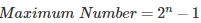# MCQs on Digital Electronics

##### Page 5 of 35. Go to page 1 2 3 4 5 6 7 8 9 10 11 12 13 14 15 16 17 18 19 20 21 22 23 24 25 26 27 28 29 30 31 32 33 34 35
01․ A + Ā is equal to
A
Ā
0
1

Logical OR of a variable and its complement is always equal to 1, i.e. A + Ā = 1.

02․ A . Ā is equal to
A
Ā
0
1

Logical AND (Intersection) of a variable and its complement is always equal to 0 i.e. A.Ā = 0. Multipication with zero is always zero.

03․ Complement of XNOR and NOR is ________ and ________ respectively.
NOR, XNOR
OR, NOR
XOR, NOR
XOR, OR

Complement of XNOR is XOR and complement of OR is NOR.

04․ A binary number system is of base ________
2
1
10
4

The radix of a binary number system is 2. Base-2 system is known as the binary system, having values as 1 and 0.

05․ Convert the binary number (1111000011110000) to hexadecimal number
1010
F0F0
7070
5050

Binary equivalent of Hexadecimal number system of F = 1111 and 0 = 0000. Binary system can be easily converted to hexadecimal by taking groups of four bits from right to left.

06․ Maximum number in decimal that can be represented by 4 bits (binary) is
4
7
15
16, where n is the number of bits. Max Number represented by 4 bits = 24 - 1 ⇒ 15.

07․ 1s Complement of 11001010 is
11001011
11001001
00110101
00110111

1's complement of a binary number can be found out by inverting each bit in the binary number system.

08․ Positive integers must be represented by
signed numbers
unsigned number
Both A and B
None of these

Positive integers are represented using unsigned numbers and negative integers are represented by signed numbers on the extreme left.

09․ 2's Complement of 10101011 is
01010101
00111100
10101011
10101100

2's Complement = 1's Complement + 1. 1's Complement of 10101011 is 01010100. 2's Complement of 10101011 is 01010101.

10․ The complement of a function A is
1
0
A
Ā

Complement or inverse of a function A is represented by Ā.

<<<34567>>>